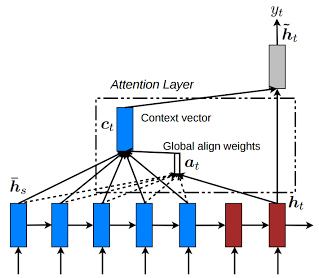Hi,
I am looking into the code about(Previous version harvardnlp/seq2seq-attn)
`local out = decoder_clones[t]:forward(decoder_input) local out_pred_idx = #out local next_state = {} table.insert(preds, out[out_pred_idx]) if opt.input_feed == 1 then table.insert(next_state, out[out_pred_idx]) end for j = 1, out_pred_idx-1 do table.insert(next_state, out[j]) end rnn_state_dec[t] = next_state end`

Here is the details of input_feed.
’-input_feed’, true,
[[Feed the context vector at each time step as additional input]]

I have a question that, the code about input_feed ，
`table.insert(next_state, out[out_pred_idx])`
means the current time step attentional vector is fed as inputs to the next time steps?
and the
`table.insert(next_state, out[j])`
means the previous time steps attentional vectors are fed as inputs to the next time steps?
But if the input_feed= False, why only the previous time steps attentional vectors are fed as inputs to the next time steps?

This is correct.

The following loop:

``````for j = 1, out_pred_idx-1 do
table.insert(next_state, out[j])
end
``````

actually prepares the next states of the LSTM, namely the cell and hidden states of each layer.

Is the `out` means the attentional hidden state (vectors) ?
So the the current time step attentional vector `out[out_pred_idx]` is the source-side context vector.
The previous time steps attentional vectors are the target hidden state.
There is a simple concatenation layer to combine the information from both target hidden state and source-side context vector to produce an attentional hidden state `out`.
In the past ,we only use the previous time steps attentional vectors.
In this Input-feeding Approach, we use the the source-side context vector as inputs to the next time steps, in other words, the whole attentional vectors(in the code).

`out` is the output of the decoder at the current timesteps. It contains all the LSTM states (cell + hidden of each layer) and the output of the attention layer which is the “context vector”.1 Like

I got it wrong.
And now I know that the `out` is the concatenation of Ct and ht ,from the image in that paper.

One more question,
In the line 459 , `decoder_clones[t]:training()`
the model training.
Then in the line 466, `local out = decoder_clones[t]:forward(decoder_input)`,got the output of the decoder.
Could you confirm my understanding?

``````decoder_clones[t]:training()